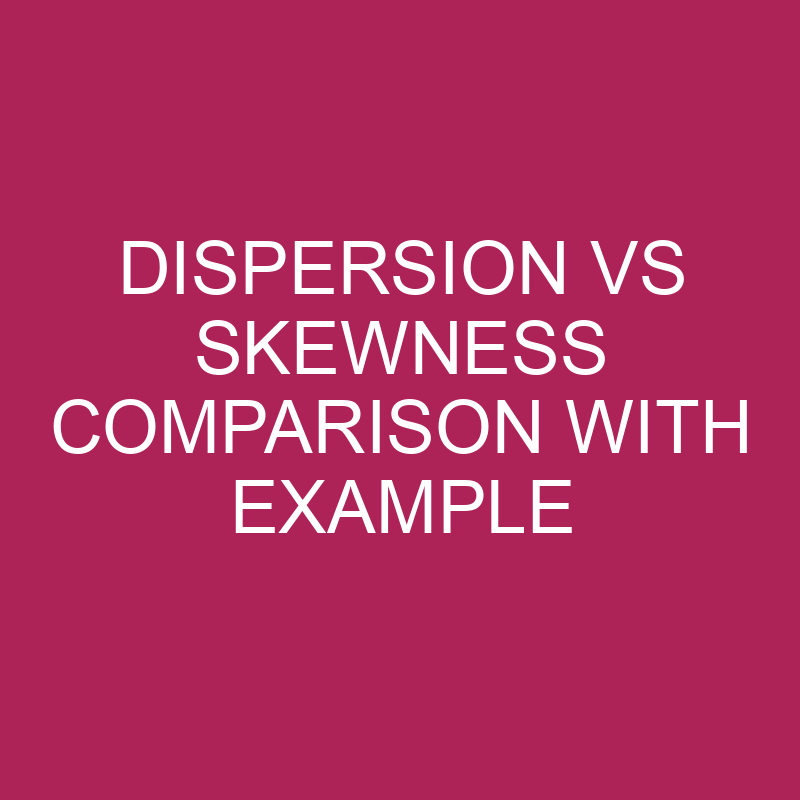Dispersion and skewness are two different measures that are used to describe the distribution of data.

## What is Dispersion?

Dispersion and skewness are both measures of the distribution of data. Dispersion refers to how spread out the data is, while skewness refers to the shape of the distribution. Dispersion can be measured using various statistics, such as range, variance, and standard deviation. These statistics give an idea of how spread out the data is around the central tendency (mean, median, or mode). The larger the dispersion, the more spread out the data is.

## Examples of Dispersion

Dispersion measures how spread out the data is. There are several measures of dispersion, including range, variance, and standard deviation.

For example, consider the following two datasets:

Dataset A: 1, 2, 3, 4, 5 Dataset B: 1, 1, 3, 5, 5

The range of dataset A is 4 (the difference between the maximum value 5 and the minimum value 1), while the range of dataset B is 4 (the difference between the maximum value 5 and the minimum value 1). However, the variance and standard deviation of dataset B are smaller than that of dataset A, indicating that the values in dataset B are less spread out than those in dataset A.

## What is Skewness?

Skewness, on the other hand, measures the asymmetry of the distribution. A distribution is said to be skewed if it is not symmetric. Skewness can be positive, negative, or zero. A positive skewness means that the tail of the distribution is longer on the right-hand side than on the left-hand side, while a negative skewness means the tail is longer on the left-hand side. Zero skewness indicates a symmetric distribution.

## Examples of Skewness

Skewness measures the asymmetry of the distribution. A distribution can be positively skewed, negatively skewed, or symmetrical.

For example, consider the following two datasets:

Dataset C: 1, 2, 3, 4, 50 Dataset D: 1, 5, 7, 8, 9

The distribution of dataset C is positively skewed because the tail of the distribution is longer on the right-hand side due to the presence of the outlier value 50. The distribution of dataset D is symmetrical as there is no skewness in the dataset.

## Pros and Cons of Dispersion

Dispersion and skewness are two important measures that can be used to describe the distribution of data. Each measure has its own advantages and disadvantages.

Pros of Dispersion:

1. Provides information about how spread out the data is around the central tendency (mean, median or mode).
2. Can be used to compare the variability of different datasets.
3. Is easily calculated using statistical formulas.

Cons of Dispersion:

1. Can be sensitive to outliers, which can significantly affect the measures of dispersion.
2. Cannot provide information about the shape or asymmetry of the distribution.
3. Does not provide any information about the individual values in the dataset.

## Pros and Cons of Skewness

Pros of Skewness:

1. Can provide information about the shape and symmetry of the distribution.
2. Can help identify outliers and influential data points.
3. Is useful in comparing the shapes of different distributions.

Cons of Skewness:

1. Can be difficult to interpret, especially when the magnitude of the skewness is small.
2. Can be influenced by the sample size.
3. Only measures one aspect of the distribution and does not provide information about the spread of the data.

## Conclusion

In summary, dispersion and skewness are both important measures that can provide valuable information about the distribution of data.

While dispersion is useful for measuring the spread of the data, skewness is useful for measuring the shape and asymmetry of the distribution. However, both measures have their own limitations and should be used in conjunction with other measures to fully understand the characteristics of a dataset.